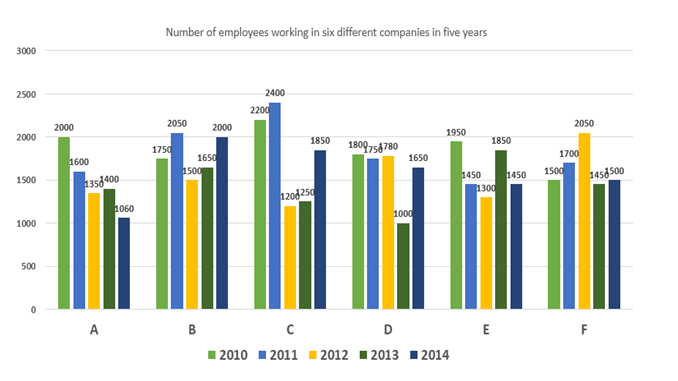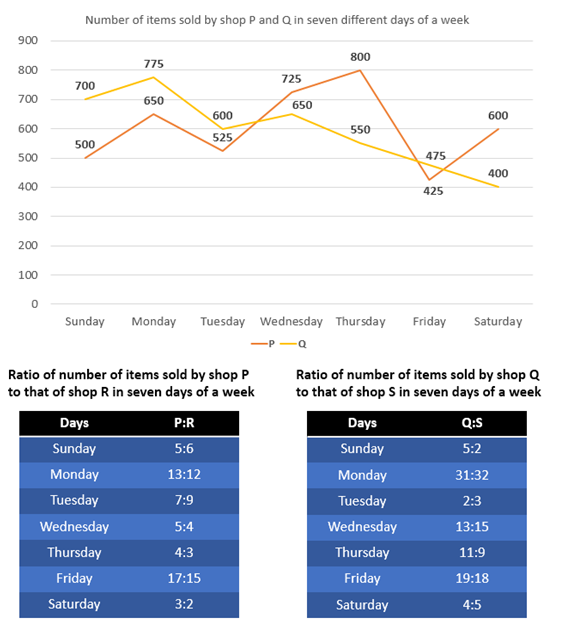# Quantitative Aptitude Questions (Data Interpretation) for SBI Clerk 2018 Day-14

## Quantitative Aptitude Questions (Data Interpretation) for SBI Clerk 2018 Day-14:

Dear Readers, SBI is conducting Online preliminary Examination for the recruitment of Clerical Cadre. preliminary Examination of SBI Clerk was scheduled from March 2018. To enrich your preparation here we have providing new series of Data Interpretation – Quantitative Aptitude Questions. Candidates those who are appearing in SBI Clerk Prelims Exam can practice these Quantitative Aptitude average questions daily and make your preparation effective.

Click “Start Quiz” to attend these Questions and view Solutions

## Daily Practice Test Schedule | Good Luck

 Topic Daily Publishing Time Daily News Papers & Editorials 8.00 AM Current Affairs Quiz 9.00 AM Logical Reasoning 10.00 AM Quantitative Aptitude “20-20” 11.00 AM Vocabulary (Based on The Hindu) 12.00 PM Static GK Quiz 1.00 PM English Language “20-20” 2.00 PM Banking Awareness Quiz 3.00 PM Reasoning Puzzles & Seating 4.00 PM Daily Current Affairs Updates 5.00 PM Data Interpretation / Application Sums (Topic Wise) 6.00 PM Reasoning Ability “20-20” 7.00 PM English Language (New Pattern Questions) 8.00 PM General / Financial Awareness Quiz 9.00 PM

Directions (Q. 1 – 5): Study the following information carefully and answer the questions given below:1) Total number of employees working in all the companies in 2010 is what percent of total number of employees working in all the companies in 2014?

1. 108.17%
2. 117.77%
3. 155.57%
4. 112.13%
5. none of these
1. Total number of employees working in C and D together in 2011 is what percent more than that of A and B together in 2013?
1. 32.16%
2. 36.06%
3. 34.32%
4. 28.42%
5. none of these
1. Find the respective ratio of total number of employees working in 2011 and that in 2012 in all the companies.
1. 355 : 321
2. 355 : 231
3. 365 : 306
4. 306 : 365
5. none of these
1. What is the difference between total number of employees working in company B in all the years together and that in D in all the years together?
1. 940
2. 930
3. 950
4. 970
5. none of these
1. Find the sum of total number of employees working in E and F in all the years together.
1. 14200
2. 16200
3. 17200
4. 15200
5. none of these

Directions (Q. 6 – 10): Study the following information carefully and answer the questions given below:1. Total number of items sold by P in the week is what percent of the total number of items sold by Q in the week?
1. 110.8%
2. 101.8%
3. 102.7%
4. 103.4%
5. none of these
1. Number of items sold by shop R in Wednesday and Thursday together is what percent more than the number of items sold by shop S in Thursday and Friday together?
1. 21.11%
2. 32.12%
3. 31.11%
4. 18.25%
5. none of these
1. Find the respective ratio of number of items sold by shop R in Sunday, Monday and Tuesday together and the number of items sold by shop S in Wednesday, Thursday and Friday together.
1. 23:21
2. 21:23
3. 22:25
4. 25:22
5. none of these
1. Find the total number of items sold by shop R in the week.
1. 3083
2. 3830
3. 3803
4. 3038
5. none of these
1. Find the difference between total number of items sold by shop Q and shop S in the week.
1. 45
2. 29
3. 20
4. 30
5. none of these

Directions (Q. 1 – 5):

Total number of employees working in all the companies in 2010

= 2000 + 1750 + 2200 + 1800 + 1950 + 1500

= 11200

Total number of employees working in all the companies in 2014

= 1060 + 2000 + 1850 + 1650 + 1450 + 1500

= 9510

Required percentage = 11200/9510 x 100 = 117.77%

Total number of employees working in C and D together in 2011 = 2400 + 1750 = 4150

Total number of employees working in A and B together in 2013 = 1400 + 1650 = 3050

Required percentage = (4150 – 3050)/3050 x 100 = 36.06%

Total number of employees working in 2011 = 1600 + 2050 + 2400 + 1750 + 1450 + 1700 = 10950

Total number of employees working in 2012 = 1350 + 1500 + 1200 + 1780 + 1300+ 2050 = 9180

Required ratio = 10950:9180 = 365 : 306

Total number of employees working in company B in all the years together

= 1750 + 2050 + 1500 + 1650 + 2000 = 8950

Total number of employees working in company D in all the years together

= 1800 + 1750 + 1780 + 1000 + 1650 = 7980

Required difference = 8950 – 7980 = 970

Required sum = 1950 + 1450 + 1300 + 1850 + 1450 + 1500 + 1700 + 2050 + 1450 + 1500 = 16200

Directions (Q. 6 – 10):

Total number of items sold by P in the week = 500 + 650 + 525 + 725 + 800 + 425 + 600 = 4225

Total number of items sold by Q in the week = 700 + 775 + 600 + 650 + 550 + 475 + 400 = 4150

Required percentage = 4225/4150 x 100 = 101.8%

Number of items sold by shop R in Wednesday and Thursday together = 4/5 x 725 + 3/4 x 800

= 580 + 600

= 1180

Number of items sold by shop S in Thursday and Friday together = 9/11 x 550 + 18/19 x 475

= 450 + 450

= 900

Required percentage = (1180 – 900)/900 x 100 = 31.11%

Number of items sold by shop R in Sunday, Monday and Tuesday together

= 6/5 x 500 + 12/13 x 650 + 9/7 x 525

= 600 + 600 + 675

= 1875

Number of items sold by shop S in Wednesday, Thursday and Friday together

= 15/13 x 650 + 9/11 x 550 + 18/19 x 475

= 750 + 450 + 450

= 1650

Required ratio = 1875:1650 = 25:22

Total number of items sold by shop R in the week

= 6/5 x 500 + 12/13 x 650 + 9/7 x 525 + 4/5 x 725 + 3/4 x 800 + 15/17 x 425 + 2/3 x 600

= 600 + 600 + 675 + 580 + 600 + 375 + 400

= 3830

Total number of items sold by Q in the week = 700 + 775 + 600 + 650 + 550 + 475 + 400 = 4150

Total number of items sold by S in the week

= 2/5 x 700 + 32/31 x 775 + 3/2 x 600 + 15/13 x 650 + 9/11 x 550 + 18/19 x 475 + 5/4 x 400

= 280 + 800 + 900 + 750 + 450 + 450 + 500

= 4130

Required difference = 4150 – 4130 = 20